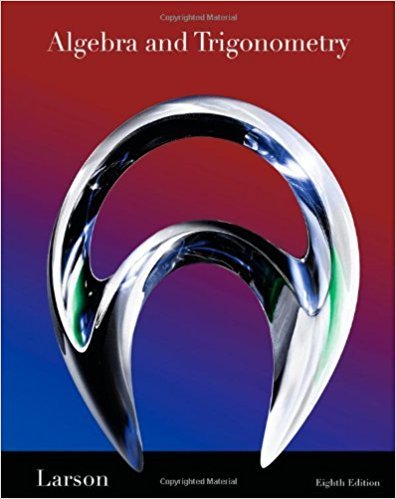×

×

# Solutions for Chapter 2.7: Inverse Functions## Full solutions for Algebra and Trigonometry | 8th Edition

ISBN: 9781439048474Solutions for Chapter 2.7: Inverse Functions

Solutions for Chapter 2.7
4 5 0 360 Reviews
25
3
##### ISBN: 9781439048474

This textbook survival guide was created for the textbook: Algebra and Trigonometry, edition: 8. Since 118 problems in chapter 2.7: Inverse Functions have been answered, more than 112882 students have viewed full step-by-step solutions from this chapter. This expansive textbook survival guide covers the following chapters and their solutions. Algebra and Trigonometry was written by and is associated to the ISBN: 9781439048474. Chapter 2.7: Inverse Functions includes 118 full step-by-step solutions.

Key Math Terms and definitions covered in this textbook
• Change of basis matrix M.

The old basis vectors v j are combinations L mij Wi of the new basis vectors. The coordinates of CI VI + ... + cnvn = dl wI + ... + dn Wn are related by d = M c. (For n = 2 set VI = mll WI +m21 W2, V2 = m12WI +m22w2.)

A sequence of steps (end of Chapter 9) to solve positive definite Ax = b by minimizing !x T Ax - x Tb over growing Krylov subspaces.

• Cyclic shift

S. Permutation with S21 = 1, S32 = 1, ... , finally SIn = 1. Its eigenvalues are the nth roots e2lrik/n of 1; eigenvectors are columns of the Fourier matrix F.

• Determinant IAI = det(A).

Defined by det I = 1, sign reversal for row exchange, and linearity in each row. Then IAI = 0 when A is singular. Also IABI = IAIIBI and

• Diagonalization

A = S-1 AS. A = eigenvalue matrix and S = eigenvector matrix of A. A must have n independent eigenvectors to make S invertible. All Ak = SA k S-I.

• Dimension of vector space

dim(V) = number of vectors in any basis for V.

• Free columns of A.

Columns without pivots; these are combinations of earlier columns.

• Hankel matrix H.

Constant along each antidiagonal; hij depends on i + j.

• Inverse matrix A-I.

Square matrix with A-I A = I and AA-l = I. No inverse if det A = 0 and rank(A) < n and Ax = 0 for a nonzero vector x. The inverses of AB and AT are B-1 A-I and (A-I)T. Cofactor formula (A-l)ij = Cji! detA.

• Kronecker product (tensor product) A ® B.

Blocks aij B, eigenvalues Ap(A)Aq(B).

• Linear transformation T.

Each vector V in the input space transforms to T (v) in the output space, and linearity requires T(cv + dw) = c T(v) + d T(w). Examples: Matrix multiplication A v, differentiation and integration in function space.

• Markov matrix M.

All mij > 0 and each column sum is 1. Largest eigenvalue A = 1. If mij > 0, the columns of Mk approach the steady state eigenvector M s = s > O.

• Norm

IIA II. The ".e 2 norm" of A is the maximum ratio II Ax II/l1x II = O"max· Then II Ax II < IIAllllxll and IIABII < IIAIIIIBII and IIA + BII < IIAII + IIBII. Frobenius norm IIAII} = L La~. The.e 1 and.e oo norms are largest column and row sums of laij I.

• Outer product uv T

= column times row = rank one matrix.

• Pascal matrix

Ps = pascal(n) = the symmetric matrix with binomial entries (i1~;2). Ps = PL Pu all contain Pascal's triangle with det = 1 (see Pascal in the index).

• Rotation matrix

R = [~ CS ] rotates the plane by () and R- 1 = RT rotates back by -(). Eigenvalues are eiO and e-iO , eigenvectors are (1, ±i). c, s = cos (), sin ().

• Semidefinite matrix A.

(Positive) semidefinite: all x T Ax > 0, all A > 0; A = any RT R.

• Special solutions to As = O.

One free variable is Si = 1, other free variables = o.

• Sum V + W of subs paces.

Space of all (v in V) + (w in W). Direct sum: V n W = to}.

• Triangle inequality II u + v II < II u II + II v II.

For matrix norms II A + B II < II A II + II B II·